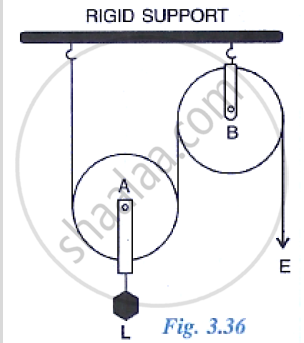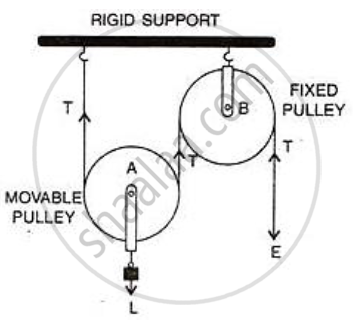Share
Notifications

View all notifications
Books Shortlist
Your shortlist is empty

# The Diagram Below Shows a Pulley Arrangement. (A) in the Diagram, Mark the Direction of Tension on Each Strand of String. (B) What is the Purpose of the Pulley B?(C) If the Tension is T, Deduce the Re - ICSE Class 10 - Physics

Login
Create free account

Forgot password?

#### Question

The diagram below shows a pulley arrangement.
(a) In the diagram, mark the direction of tension on each strand of string.
(b) What is the purpose of the pulley B?

(c) If the tension is T, Deduce the relation between T and E.
(d) What is the velocity ratio of the arrangement?
(e) Assuming that the efficiency of the system is 100%, What is the mechanical advantage?#### Solution

(a)(b) The fixed pulley B is used to change the direction of effort to be applied from upward to           downward.
(c) The effort E balances the tension T at the free end, so E=T
(d) The velocity ratio of this arrangement is 2.
(e) The mechanical advantage is 2 for this system (if efficiency is 100%).

Is there an error in this question or solution?

#### APPEARS IN

Solution The Diagram Below Shows a Pulley Arrangement. (A) in the Diagram, Mark the Direction of Tension on Each Strand of String. (B) What is the Purpose of the Pulley B?(C) If the Tension is T, Deduce the Re Concept: Concept of Pulley System.
S